# Mean State

Period Mean (original grids) [Watt m-2]
Model Period Mean (intersection) [Watt m-2]
Model Period Mean (complement) [Watt m-2]
Benchmark Period Mean (intersection) [Watt m-2]
Benchmark Period Mean (complement) [Watt m-2]
Bias [Watt m-2]
RMSE [Watt m-2]
Phase Shift [months]
Bias Score 
RMSE Score 
Seasonal Cycle Score 
Spatial Distribution Score 
Interannual Variability Score 
Overall Score 
Benchmark [-] 453.
CRUNCEPv7 [-] 462. 462. 0.00 453. 460. 10.0 15.8 1.71 0.36 0.38 0.75 0.99 0.72 0.60
GSWP3v1 [-] 462. 462. 0.00 453. 460. 9.34 15.7 1.72 0.34 0.37 0.75 0.99 0.71 0.59
WATCH [-] 456. 456. 0.00 452. 460. 5.05 14.3 2.00 0.43 0.35 0.70 0.99 0.74 0.59
Period Mean (original grids) [Watt m-2]
Model Period Mean (intersection) [Watt m-2]
Model Period Mean (complement) [Watt m-2]
Benchmark Period Mean (intersection) [Watt m-2]
Benchmark Period Mean (complement) [Watt m-2]
Bias [Watt m-2]
RMSE [Watt m-2]
Phase Shift [months]
Bias Score 
RMSE Score 
Seasonal Cycle Score 
Spatial Distribution Score 
Interannual Variability Score 
Overall Score 
Benchmark [-] 471.
CRUNCEPv7 [-] 485. 485. 0.00 471. 456. 13.4 23.9 0.875 0.50 0.47 0.93 1.0 0.76 0.69
GSWP3v1 [-] 481. 482. 0.00 471. 456. 10.1 20.9 0.713 0.56 0.50 0.95 0.95 0.71 0.69
WATCH [-] 466. 466. 0.00 470. 455. -3.27 21.8 1.07 0.65 0.41 0.90 1.0 0.76 0.69
Period Mean (original grids) [Watt m-2]
Model Period Mean (intersection) [Watt m-2]
Model Period Mean (complement) [Watt m-2]
Benchmark Period Mean (intersection) [Watt m-2]
Benchmark Period Mean (complement) [Watt m-2]
Bias [Watt m-2]
RMSE [Watt m-2]
Phase Shift [months]
Bias Score 
RMSE Score 
Seasonal Cycle Score 
Spatial Distribution Score 
Interannual Variability Score 
Overall Score 
Benchmark [-] 443.
CRUNCEPv7 [-] 450. 449. 0.00 443. 444. 6.37 18.0 0.975 0.56 0.51 0.89 0.96 0.64 0.68
GSWP3v1 [-] 451. 451. 0.00 443. 444. 7.63 17.9 1.14 0.57 0.49 0.85 0.99 0.61 0.67
WATCH [-] 443. 443. 0.00 441. 444. 2.24 16.3 1.33 0.61 0.49 0.83 0.97 0.72 0.68
Period Mean (original grids) [Watt m-2]
Model Period Mean (intersection) [Watt m-2]
Model Period Mean (complement) [Watt m-2]
Benchmark Period Mean (intersection) [Watt m-2]
Benchmark Period Mean (complement) [Watt m-2]
Bias [Watt m-2]
RMSE [Watt m-2]
Phase Shift [months]
Bias Score 
RMSE Score 
Seasonal Cycle Score 
Spatial Distribution Score 
Interannual Variability Score 
Overall Score 
Benchmark [-] 276.
CRUNCEPv7 [-] 265. 265. 0.00 275. 292. -7.59 19.0 0.0691 0.86 0.78 1.0 0.97 0.77 0.86
GSWP3v1 [-] 275. 275. 0.00 275. 292. 3.64 16.0 0.0557 0.90 0.80 1.0 0.99 0.79 0.88
WATCH [-] 266. 267. 0.00 274. 291. -5.33 19.5 0.0661 0.89 0.76 1.0 0.98 0.85 0.87
Period Mean (original grids) [Watt m-2]
Model Period Mean (intersection) [Watt m-2]
Model Period Mean (complement) [Watt m-2]
Benchmark Period Mean (intersection) [Watt m-2]
Benchmark Period Mean (complement) [Watt m-2]
Bias [Watt m-2]
RMSE [Watt m-2]
Phase Shift [months]
Bias Score 
RMSE Score 
Seasonal Cycle Score 
Spatial Distribution Score 
Interannual Variability Score 
Overall Score 
Benchmark [-] 456.
CRUNCEPv7 [-] 456. 456. 0.00 453. 465. 4.81 10.5 2.03 0.31 0.38 0.68 0.99 0.67 0.57
GSWP3v1 [-] 458. 458. 0.00 453. 465. 5.84 10.1 1.76 0.31 0.41 0.73 0.93 0.72 0.59
WATCH [-] 456. 456. 0.00 453. 464. 4.59 10.9 2.10 0.29 0.36 0.68 0.98 0.77 0.57
Period Mean (original grids) [Watt m-2]
Model Period Mean (intersection) [Watt m-2]
Model Period Mean (complement) [Watt m-2]
Benchmark Period Mean (intersection) [Watt m-2]
Benchmark Period Mean (complement) [Watt m-2]
Bias [Watt m-2]
RMSE [Watt m-2]
Phase Shift [months]
Bias Score 
RMSE Score 
Seasonal Cycle Score 
Spatial Distribution Score 
Interannual Variability Score 
Overall Score 
Benchmark [-] 306.
CRUNCEPv7 [-] 309. 309. 0.00 306. 329. 3.73 11.7 0.0258 0.92 0.87 1.0 0.98 0.89 0.92
GSWP3v1 [-] 316. 315. 0.00 306. 329. 10.1 14.8 0.0215 0.86 0.86 1.0 1.0 0.89 0.91
WATCH [-] 309. 309. 0.00 305. 329. 4.00 12.8 0.0322 0.91 0.85 1.0 0.97 0.90 0.92
Period Mean (original grids) [Watt m-2]
Model Period Mean (intersection) [Watt m-2]
Model Period Mean (complement) [Watt m-2]
Benchmark Period Mean (intersection) [Watt m-2]
Benchmark Period Mean (complement) [Watt m-2]
Bias [Watt m-2]
RMSE [Watt m-2]
Phase Shift [months]
Bias Score 
RMSE Score 
Seasonal Cycle Score 
Spatial Distribution Score 
Interannual Variability Score 
Overall Score 
Benchmark [-] 362.
CRUNCEPv7 [-] 361. 360. 0.00 361. 395. 1.74 14.5 0.198 0.87 0.83 0.99 1.0 0.75 0.88
GSWP3v1 [-] 364. 363. 0.00 361. 395. 4.75 15.4 0.151 0.86 0.82 0.99 0.99 0.73 0.87
WATCH [-] 356. 356. 0.00 359. 395. -1.00 16.0 0.148 0.86 0.81 0.99 1.0 0.81 0.88
Period Mean (original grids) [Watt m-2]
Model Period Mean (intersection) [Watt m-2]
Model Period Mean (complement) [Watt m-2]
Benchmark Period Mean (intersection) [Watt m-2]
Benchmark Period Mean (complement) [Watt m-2]
Bias [Watt m-2]
RMSE [Watt m-2]
Phase Shift [months]
Bias Score 
RMSE Score 
Seasonal Cycle Score 
Spatial Distribution Score 
Interannual Variability Score 
Overall Score 
Benchmark [-] 450.
CRUNCEPv7 [-] 457. 458. 0.00 450. 463. 10.2 20.0 0.673 0.56 0.57 0.94 0.99 0.65 0.71
GSWP3v1 [-] 452. 452. 0.00 450. 463. 4.66 17.7 0.767 0.65 0.56 0.92 1.0 0.63 0.72
WATCH [-] 449. 450. 0.00 448. 463. 3.43 19.5 0.794 0.64 0.52 0.92 1.0 0.66 0.71
Period Mean (original grids) [Watt m-2]
Model Period Mean (intersection) [Watt m-2]
Model Period Mean (complement) [Watt m-2]
Benchmark Period Mean (intersection) [Watt m-2]
Benchmark Period Mean (complement) [Watt m-2]
Bias [Watt m-2]
RMSE [Watt m-2]
Phase Shift [months]
Bias Score 
RMSE Score 
Seasonal Cycle Score 
Spatial Distribution Score 
Interannual Variability Score 
Overall Score 
Benchmark [-] 275.
CRUNCEPv7 [-] 273. 273. 0.00 275. 280. -1.59 15.1 0.0657 0.92 0.79 1.0 0.98 0.85 0.89
GSWP3v1 [-] 282. 282. 0.00 275. 280. 7.91 15.3 0.0355 0.87 0.82 1.0 1.0 0.85 0.89
WATCH [-] 275. 276. 0.00 274. 280. 1.50 15.6 0.0482 0.93 0.79 1.0 0.99 0.90 0.90
Period Mean (original grids) [Watt m-2]
Model Period Mean (intersection) [Watt m-2]
Model Period Mean (complement) [Watt m-2]
Benchmark Period Mean (intersection) [Watt m-2]
Benchmark Period Mean (complement) [Watt m-2]
Bias [Watt m-2]
RMSE [Watt m-2]
Phase Shift [months]
Bias Score 
RMSE Score 
Seasonal Cycle Score 
Spatial Distribution Score 
Interannual Variability Score 
Overall Score 
Benchmark [-] 448.
CRUNCEPv7 [-] 450. 450. 0.00 450. 423. -2.94 14.0 0.347 0.78 0.71 0.97 1.0 0.74 0.82
GSWP3v1 [-] 449. 450. 0.00 450. 423. -3.08 14.6 0.337 0.78 0.71 0.97 0.99 0.70 0.81
WATCH [-] 445. 446. 0.00 448. 422. -5.01 16.1 0.356 0.76 0.68 0.97 1.0 0.71 0.80
Period Mean (original grids) [Watt m-2]
Model Period Mean (intersection) [Watt m-2]
Model Period Mean (complement) [Watt m-2]
Benchmark Period Mean (intersection) [Watt m-2]
Benchmark Period Mean (complement) [Watt m-2]
Bias [Watt m-2]
RMSE [Watt m-2]
Phase Shift [months]
Bias Score 
RMSE Score 
Seasonal Cycle Score 
Spatial Distribution Score 
Interannual Variability Score 
Overall Score 
Benchmark [-] 364.
CRUNCEPv7 [-] 361. 361. 0.00 362. 385. 1.58 10.8 0.513 0.86 0.81 0.97 1.0 0.83 0.88
GSWP3v1 [-] 363. 363. 0.00 362. 385. 3.66 10.8 0.476 0.86 0.81 0.97 0.99 0.83 0.88
WATCH [-] 357. 357. 0.00 361. 384. -0.690 12.4 0.403 0.84 0.79 0.97 0.99 0.85 0.87
Period Mean (original grids) [Watt m-2]
Model Period Mean (intersection) [Watt m-2]
Model Period Mean (complement) [Watt m-2]
Benchmark Period Mean (intersection) [Watt m-2]
Benchmark Period Mean (complement) [Watt m-2]
Bias [Watt m-2]
RMSE [Watt m-2]
Phase Shift [months]
Bias Score 
RMSE Score 
Seasonal Cycle Score 
Spatial Distribution Score 
Interannual Variability Score 
Overall Score 
Benchmark [-] 397.
CRUNCEPv7 [-] 377. 377. 0.00 376. 406. 1.88 16.1 0.535 0.71 0.67 0.94 1.0 0.70 0.78
GSWP3v1 [-] 380. 380. 0.00 376. 406. 4.60 16.3 0.517 0.70 0.68 0.94 1.0 0.74 0.79
WATCH [-] 373. 373. 0.00 375. 406. -0.348 17.4 0.599 0.71 0.66 0.93 1.0 0.78 0.79
Period Mean (original grids) [Watt m-2]
Model Period Mean (intersection) [Watt m-2]
Model Period Mean (complement) [Watt m-2]
Benchmark Period Mean (intersection) [Watt m-2]
Benchmark Period Mean (complement) [Watt m-2]
Bias [Watt m-2]
RMSE [Watt m-2]
Phase Shift [months]
Bias Score 
RMSE Score 
Seasonal Cycle Score 
Spatial Distribution Score 
Interannual Variability Score 
Overall Score 
Benchmark [-] 464.
CRUNCEPv7 [-] 454. 454. 0.00 465. 441. -11.1 21.4 0.446 0.75 0.74 0.96 0.99 0.65 0.81
GSWP3v1 [-] 450. 449. 0.00 465. 441. -15.4 24.1 0.443 0.71 0.73 0.97 0.97 0.64 0.79
WATCH [-] 437. 436. 0.00 462. 441. -25.2 32.1 0.507 0.60 0.72 0.96 0.96 0.67 0.77
Period Mean (original grids) [Watt m-2]
Model Period Mean (intersection) [Watt m-2]
Model Period Mean (complement) [Watt m-2]
Benchmark Period Mean (intersection) [Watt m-2]
Benchmark Period Mean (complement) [Watt m-2]
Bias [Watt m-2]
RMSE [Watt m-2]
Phase Shift [months]
Bias Score 
RMSE Score 
Seasonal Cycle Score 
Spatial Distribution Score 
Interannual Variability Score 
Overall Score 
Benchmark [-] 278.
CRUNCEPv7 [-] 277. 276. 0.00 277. 289. -1.61 14.2 0.0692 0.90 0.81 1.0 0.97 0.78 0.88
GSWP3v1 [-] 285. 285. 0.00 277. 289. 8.39 14.5 0.0541 0.86 0.83 1.0 0.99 0.82 0.89
WATCH [-] 280. 280. 0.00 277. 288. 2.87 14.3 0.0516 0.90 0.80 1.0 0.98 0.88 0.89
Period Mean (original grids) [Watt m-2]
Model Period Mean (intersection) [Watt m-2]
Model Period Mean (complement) [Watt m-2]
Benchmark Period Mean (intersection) [Watt m-2]
Benchmark Period Mean (complement) [Watt m-2]
Bias [Watt m-2]
RMSE [Watt m-2]
Phase Shift [months]
Bias Score 
RMSE Score 
Seasonal Cycle Score 
Spatial Distribution Score 
Interannual Variability Score 
Overall Score 
Benchmark [-] 444.
CRUNCEPv7 [-] 445. 445. 0.00 442. 455. 6.86 15.5 0.625 0.53 0.58 0.94 0.93 0.73 0.71
GSWP3v1 [-] 446. 446. 0.00 442. 455. 7.88 14.4 0.674 0.55 0.58 0.93 0.99 0.72 0.73
WATCH [-] 445. 445. 0.00 441. 455. 6.99 16.2 0.839 0.55 0.53 0.91 0.97 0.76 0.71
Period Mean (original grids) [Watt m-2]
Model Period Mean (intersection) [Watt m-2]
Model Period Mean (complement) [Watt m-2]
Benchmark Period Mean (intersection) [Watt m-2]
Benchmark Period Mean (complement) [Watt m-2]
Bias [Watt m-2]
RMSE [Watt m-2]
Phase Shift [months]
Bias Score 
RMSE Score 
Seasonal Cycle Score 
Spatial Distribution Score 
Interannual Variability Score 
Overall Score 
Benchmark [-] 386.
CRUNCEPv7 [-] 387. 387. 0.00 384. 429. 5.60 10.2 0.275 0.87 0.85 0.98 1.0 0.89 0.91
GSWP3v1 [-] 390. 390. 0.00 384. 429. 8.58 11.9 0.176 0.83 0.86 0.99 1.0 0.86 0.90
WATCH [-] 383. 383. 0.00 383. 429. 1.98 9.68 0.148 0.90 0.84 0.99 1.0 0.90 0.91
Period Mean (original grids) [Watt m-2]
Model Period Mean (intersection) [Watt m-2]
Model Period Mean (complement) [Watt m-2]
Benchmark Period Mean (intersection) [Watt m-2]
Benchmark Period Mean (complement) [Watt m-2]
Bias [Watt m-2]
RMSE [Watt m-2]
Phase Shift [months]
Bias Score 
RMSE Score 
Seasonal Cycle Score 
Spatial Distribution Score 
Interannual Variability Score 
Overall Score 
Benchmark [-] 319.
CRUNCEPv7 [-] 322. 322. 0.00 318. 343. 4.63 11.7 0.170 0.89 0.84 0.99 1.0 0.84 0.90
GSWP3v1 [-] 327. 327. 0.00 318. 343. 10.1 14.6 0.138 0.83 0.84 0.99 0.99 0.84 0.89
WATCH [-] 322. 322. 0.00 318. 342. 5.68 12.7 0.171 0.88 0.83 0.99 1.0 0.89 0.90
Period Mean (original grids) [Watt m-2]
Model Period Mean (intersection) [Watt m-2]
Model Period Mean (complement) [Watt m-2]
Benchmark Period Mean (intersection) [Watt m-2]
Benchmark Period Mean (complement) [Watt m-2]
Bias [Watt m-2]
RMSE [Watt m-2]
Phase Shift [months]
Bias Score 
RMSE Score 
Seasonal Cycle Score 
Spatial Distribution Score 
Interannual Variability Score 
Overall Score 
Benchmark [-] 376.
CRUNCEPv7 [-] 377. 378. 0.00 376. 379. 2.07 14.8 0.131 0.86 0.81 0.99 1.0 0.77 0.88
GSWP3v1 [-] 380. 381. 0.00 376. 379. 5.26 16.3 0.108 0.85 0.80 0.99 0.98 0.71 0.86
WATCH [-] 375. 376. 0.00 374. 379. 2.16 16.9 0.183 0.85 0.79 0.99 1.0 0.79 0.87
Period Mean (original grids) [Watt m-2]
Model Period Mean (intersection) [Watt m-2]
Model Period Mean (complement) [Watt m-2]
Benchmark Period Mean (intersection) [Watt m-2]
Benchmark Period Mean (complement) [Watt m-2]
Bias [Watt m-2]
RMSE [Watt m-2]
Phase Shift [months]
Bias Score 
RMSE Score 
Seasonal Cycle Score 
Spatial Distribution Score 
Interannual Variability Score 
Overall Score 
Benchmark [-] 449.
CRUNCEPv7 [-] 455. 455. 0.00 449. 458. 6.39 13.2 1.15 0.44 0.40 0.83 0.86 0.68 0.61
GSWP3v1 [-] 457. 457. 0.00 449. 458. 7.86 13.4 1.25 0.42 0.40 0.82 0.93 0.63 0.60
WATCH [-] 453. 453. 0.00 449. 458. 3.99 13.6 1.83 0.50 0.37 0.73 0.92 0.69 0.60
Period Mean (original grids) [Watt m-2]
Model Period Mean (intersection) [Watt m-2]
Model Period Mean (complement) [Watt m-2]
Benchmark Period Mean (intersection) [Watt m-2]
Benchmark Period Mean (complement) [Watt m-2]
Bias [Watt m-2]
RMSE [Watt m-2]
Phase Shift [months]
Bias Score 
RMSE Score 
Seasonal Cycle Score 
Spatial Distribution Score 
Interannual Variability Score 
Overall Score 
Benchmark [-] 440.
CRUNCEPv7 [-] 447. 447. 0.00 440. 430. 6.01 12.7 0.806 0.68 0.60 0.90 0.98 0.77 0.76
GSWP3v1 [-] 448. 448. 0.00 440. 430. 6.99 12.9 0.794 0.66 0.60 0.89 0.98 0.73 0.75
WATCH [-] 441. 441. 0.00 439. 429. 1.49 11.8 0.917 0.71 0.59 0.88 0.97 0.78 0.75
Period Mean (original grids) [Watt m-2]
Model Period Mean (intersection) [Watt m-2]
Model Period Mean (complement) [Watt m-2]
Benchmark Period Mean (intersection) [Watt m-2]
Benchmark Period Mean (complement) [Watt m-2]
Bias [Watt m-2]
RMSE [Watt m-2]
Phase Shift [months]
Bias Score 
RMSE Score 
Seasonal Cycle Score 
Spatial Distribution Score 
Interannual Variability Score 
Overall Score 
Benchmark [-] 387.
CRUNCEPv7 [-] 383. 382. 0.00 386. 394. -2.95 18.3 0.590 0.61 0.63 0.94 1.0 0.72 0.75
GSWP3v1 [-] 387. 386. 0.00 386. 394. 0.770 16.4 0.562 0.64 0.63 0.94 1.0 0.71 0.76
WATCH [-] 387. 386. 0.00 385. 394. 1.83 16.9 0.589 0.63 0.60 0.93 1.0 0.75 0.75

# Temporally integrated period mean

BENCHMARK MEAN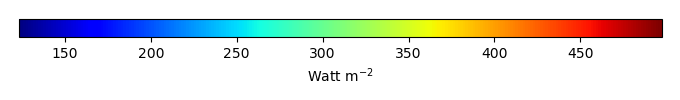MODEL MEANBIAS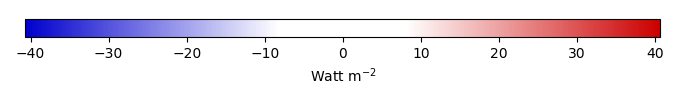BIAS SCORERMSE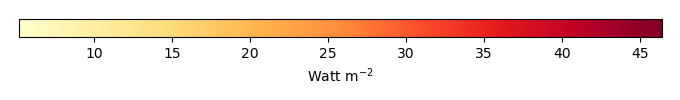RMSE SCOREBENCHMARK INTERANNUAL VARIABILITY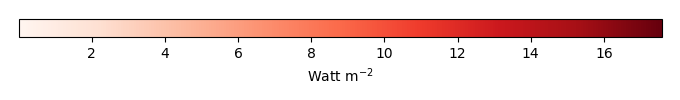MODEL INTERANNUAL VARIABILITYINTERANNUAL VARIABILITY SCOREBENCHMARK MAX MONTHMODEL MAX MONTHDIFFERENCE IN MAX MONTH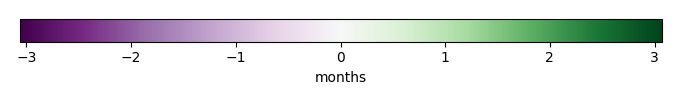SEASONAL CYCLE SCORESPATIAL TAYLOR DIAGRAMMODEL COLORS# Spatially integrated regional mean

MODEL COLORSREGIONAL MEANANNUAL CYCLEMONTHLY ANOMALYANNUAL CYCLE# All Models

BenchmarkCRUNCEPv7GSWP3v1WATCH# Data Information

creation_date: Mon Jun 30 23:35:20 PDT 2014

source_file: This product is generated from monthly 1 degree GEWEX SRB Radiation observations